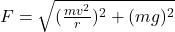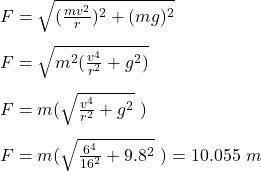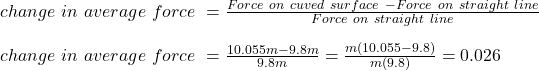## For sprinters running at 6 m/s around a curved track of radius 16 m, how much greater (as a percentage) is the average total force on their

Question

For sprinters running at 6 m/s around a curved track of radius 16 m, how much greater (as a percentage) is the average total force on their feet compared to when they are running in a straight line

in progress 0
3 weeks 2021-08-31T14:16:50+00:00 2 Answers 0 views 0

The percentage change in the average total force is 2.6%

Explanation:

The average force on the sprinters on curved track is due to centripetal force and gravity which is given as ;where;

m is the mass of each sprinter

v is the velocity of each sprinter = 6 m/s

r is the radius of the curved track = 16 m

g is the acceleration = 9.8

Substitute these values into above equation;The average force on the sprinters on straight line is affected by gravity and it is given as;

F = mg = 9.8 mPercentage change in the average total force = 0.026 x 100 = 2.6%

2. Given Information:

Sprinter speed = v = 6 m/s

Track radius = r = 16 m

Required Information:

Ratio of force on curved track vs straight track = ?

Fc – Fs/Fs = 2.60 %

Explanation:

Let Fs denotes force in straight track and Fc denotes force in curved track

When a sprinter runs on a straight track the the only force acting on his feet is the force of gravity which is given by

Fs = mg

Fs = 9.8m

When a sprinter runs on a curved track there are two forces acting on his feet, one is force of gravity and the other is inward directed friction force caused by the centripetal acceleration which can be written as

Fc = mg + mv²/r

Fc = m(g + v²/r)

The net force is the vector sum of these two forces

Fc² = (mg)² + (v²/r)²

Take square root on both sides

√Fc² = √(m²g² + (v²/r)²)

Fc = m√(g² + (v²/r)²)

Substitute the given values

Fc = m√(9.8² + (6²/16)²)

Fc = 10.055m

Now lets compare Fc with Fs

Fc – Fs/Fs = 10.055m – 9.8/9.8m

Fc – Fs/Fs = 0.026*100%

Fc – Fs/Fs = 2.60 %

Analysis:

Therefore, the results are exactly as expected, a greater force is required in the case of curved track as compared to straight track. It also explains why the runners are slowed down in curved tracks. In order to maintain the same speed as on the straight track, the runners must exert more force.